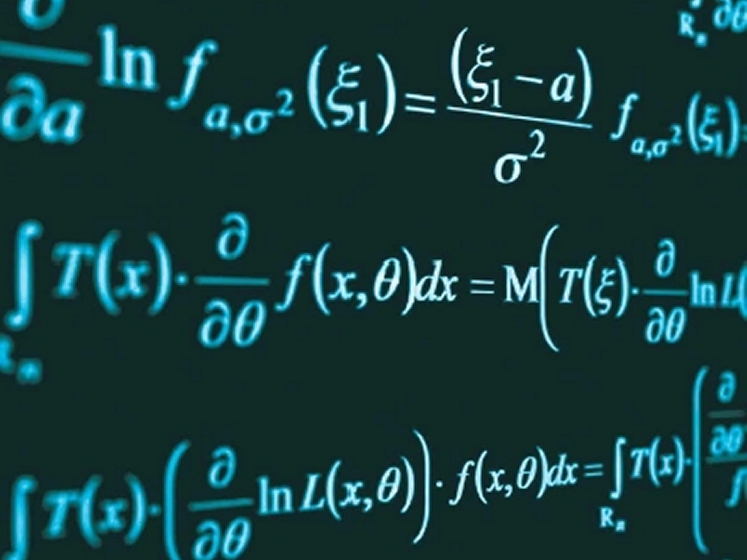Education

Different Areas of MathematicsMathematics is an important subject that tries to formalize logic. It is almost used in all professions. It helps in the development of science, technology, economics, etc. It is all about reasoning and the logic tries to practice the symbols. It improves the living standard of every person. Mathematics deals with the study of numbers, shapes and patterns. The areas of study in mathematics include numbers, shapes, change and structure.

Numbers

The numbers deal with how things can be counted in terms of quantities. Some of the areas that cover the Number System concepts are

• Natural numbers
• Real Numbers
• Complex Numbers
• Rational Numbers
• Integers
• Arithmetic Operations
• Arithmetic Relations
• Cardinal Numbers
• Functions

Shapes

Geometry is an important branch of mathematics that deals with the study of shapes, sizes, position and dimensions of the objects. In geometry, the shape of the object can be studied in terms of two-dimensional (2D) shapes and three-dimensional (3D) shapes. For any 2D shapes, the area and perimeter can be determined and the studies of 2D shapes are called “plane geometry”. For 3D shapes, the surface area and the volume can be found and the studies of 3D shapes are called “solid geometry”. For example, a triangle is a 2D shape; it is easy to find the area and perimeter of a triangle when the parameters are given. Some other area that deals with the shape of the object includes

• Trigonometry
• Fractal Geometry
• Differential Geometry
• Topology

Change

To study the rate of change and the way things changes can be studied under the following concepts like

• Calculus – Differentiation and Integration
• Differential Equations
• Vector Calculus

Structure

Algebra is an important concept in mathematics that uses the variables to represent the value of any logical sentence in terms of equations. Some of the inequalities in algebra help to draw graphs in many fields like constructions, business, and accounting and most importantly in surveying. The areas of study in mathematics are

• Number Theory
• Linear Algebra
• Graph Theory

All the concepts in mathematics are related to daily life activities. It literally Shapes our lives. Most of the theorems from other disciplines are evolved from mathematics and formulas like an area of a circle, triangle, square, the volume of the cylinder, a cone is often used.

Subscribe to BYJU’S YouTube channel to learn more concepts in mathematics and also download BYJU’S – The Learning App for interactive videos.Finance

Finance

Finance

Finance

Finance

Games

Games

Games

Entertainment